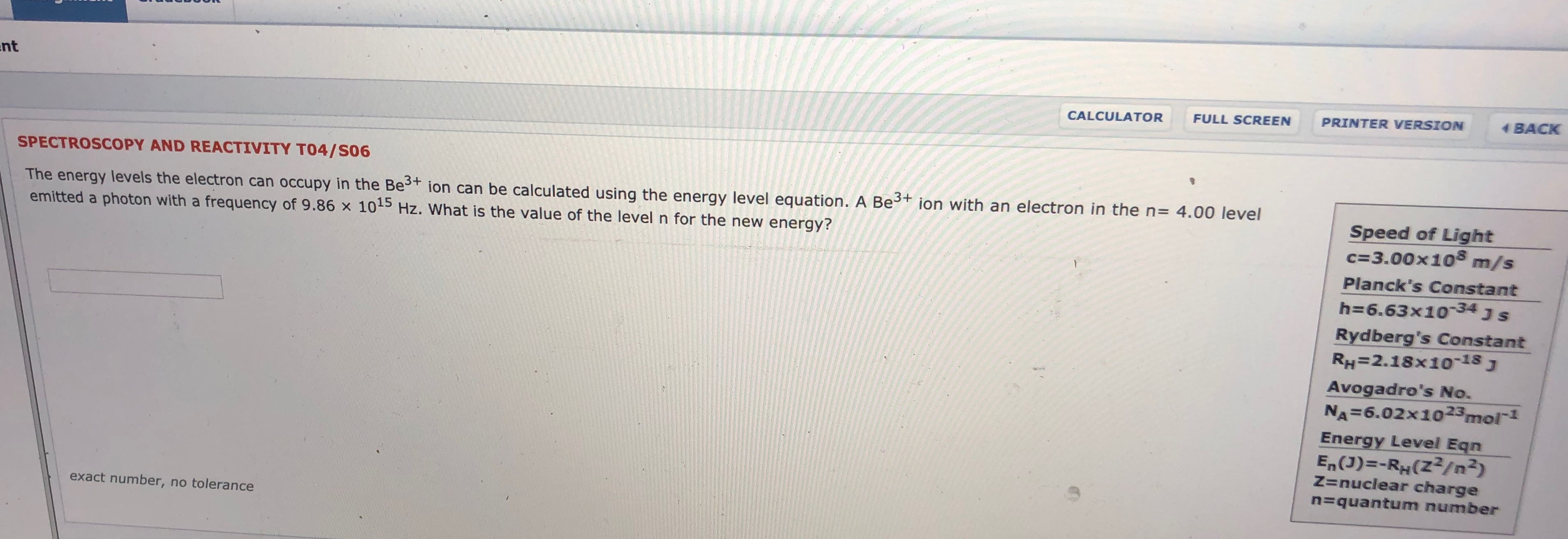# ntBACKPRINTER VERSIONFULL SCREENCALCULATORSPECTROSCOPY AND REACTIVITY TO4/S06The energy levels the electron can occupy in the Bet ion can be calculated using the energy level equation. A Beemitted a photon with a frequency of 9.86 x 105 Hz. What is the value of the level n for the new energy?ion with an electron in the n4.00 levelSpeed of Lightc 3.00x108m/sPlanck's Constanth 6.63x1034 J SRydberg's ConstantRH=2.18x1o-18JAvogadro's No.NA 6.02x1023mol1Energy Level EqnEn(J)-RH(Z2/n2)Z=nuclear charge=quantum numberexact number, no tolerance

Question
3 viewshelp_outlineImage Transcriptionclosent BACK PRINTER VERSION FULL SCREEN CALCULATOR SPECTROSCOPY AND REACTIVITY TO4/S06 The energy levels the electron can occupy in the Bet ion can be calculated using the energy level equation. A Be emitted a photon with a frequency of 9.86 x 105 Hz. What is the value of the level n for the new energy? ion with an electron in the n 4.00 level Speed of Light c 3.00x108 m/s Planck's Constant h 6.63x1034 J S Rydberg's Constant RH=2.18x1o-18J Avogadro's No. NA 6.02x1023mol1 Energy Level Eqn En(J)-RH(Z2/n2) Z=nuclear charge =quantum number exact number, no tolerance fullscreen
check_circle

Step 1

Given information:

Energy level for Be3+ ion (n)= 4

Frequency for Be3+ ion = 9.86×1015 Hz

Step 2

The energy can be expressed as the product of a constant that is plank’s constant(h) and frequency(ν). The mathematical expression for this is given as:

Step 3

Thus, for given frequency with energy leve...

### Want to see the full answer?

See Solution

#### Want to see this answer and more?

Solutions are written by subject experts who are available 24/7. Questions are typically answered within 1 hour.*

See Solution
*Response times may vary by subject and question.
Tagged in

### Atomic structure